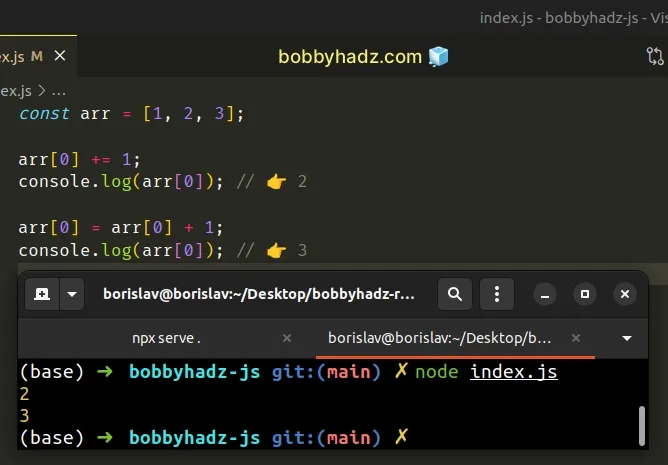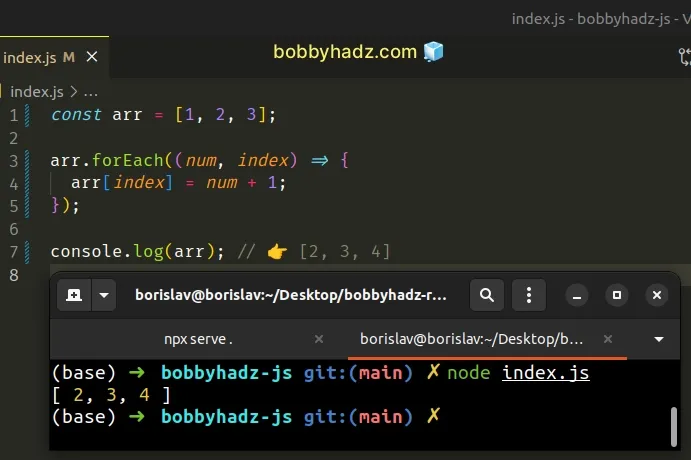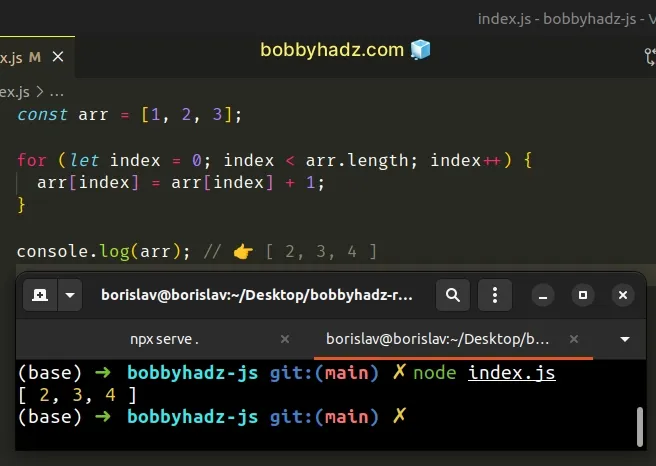# Increment the Values in an Array using JavaScriptLast updated: Jan 8, 2023
3 min## #Increment the Values in an Array in JavaScript

To increment the values in an array:

1. Use the `Array.map()` method to iterate over the array.
2. Increment each value and return the result.
3. The `map()` method will return a new array containing the incremented values.
index.js
```Copied!```const arr = [1, 2, 3];

const newArr = arr.map(num => num + 1);
console.log(newArr); // 👉️ [2, 3, 4]
``````The function we passed to the Array.map() method gets called with each element in the array.

On each iteration, we add `1` to the current number and return the result.

The `map()` method returns a new array containing the values returned from the callback function.

The `Array.map()` method doesn't mutate the original array, it returns a new array.

## #Creating an incrementing array in JavaScript

If you need to create an incrementing array, use the `Array.from()` method.

index.js
```Copied!```const arr1 = Array.from(Array(5), (_, index) => index);
console.log(arr1); // 👉️ [ 0, 1, 2, 3, 4 ]

const arr2 = Array.from(Array(5), (_, index) => index + 1);
console.log(arr2); // 👉️ [ 1, 2, 3, 4, 5 ]
``````The function we passed to the `Array.from()` method is a `map()` function, just like in the previous example.

You can use the index to create an incrementing array.

## #Increment specific values in an array in JavaScript

If you need to increment a specific value, access the array at an index and increment the value.

index.js
```Copied!```const arr = [1, 2, 3];

arr += 1;
console.log(arr); // 👉️ 2

arr = arr + 1;
console.log(arr); // 👉️ 3
``````The addition assignment (+=) operator is a shorthand for `myVariable = myVariable + value`.

JavaScript indexes are zero-based, so the first element in the array has an index of `0`, and the last element has an index of `array.length - 1`.

When you access the array at the specific index and assign a new value to it, you mutate the original array and change its value in place.

Note that when using the addition assignment (+=) operator with primitives like strings or numbers, the variable has to be declared using the `let` keyword.

index.js
```Copied!```let a = 1;

a += 5;
console.log(a); // 👉️ 6

// 👇️ same as above
a = a + 5;
console.log(a) // 👉️ 11

const b = 1;

// ⛔️ SyntaxError
b ++ 5;
``````

When using the addition assignment (+=) operator with primitives, we reassign the variable.

This is not the case with arrays or objects, where we change the value of a specific element without reassigning the actual variable.

Alternatively, you can use the Array.forEach method.

## #Increment the values in an array using `forEach()`

This is a two-step process:

1. Use the `Array.forEach()` method to iterate over the array.
2. On each iteration, increment the element at the current index by `1`.
index.js
```Copied!```const arr = [1, 2, 3];

arr.forEach((num, index) => {
arr[index] = num + 1;
});

console.log(arr); // 👉️ [2, 3, 4]
``````The function we passed to the Array.forEach method gets called with each element in the array.

We access the element at the current index and increment its value by `1`.

The `forEach()` method returns `undefined`, so we have to perform some kind of mutation to persist the state.

You can also use a basic `for` loop.

## #Increment the values in an array using a `for` loop

This is a two-step process:

1. Use a `for` loop to iterate over the array.
2. On each iteration, increment the element at the current index by `1`.
index.js
```Copied!```const arr = [1, 2, 3];

for (let index = 0; index < arr.length; index++) {
arr[index] = arr[index] + 1;
}

console.log(arr); // 👉️ [ 2, 3, 4 ]
``````The code sample is very similar to the previous one.

However, instead of using the `Array.forEach()` method, we used a basic `for` loop to iterate over the array.